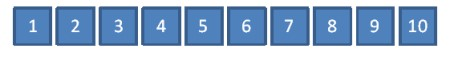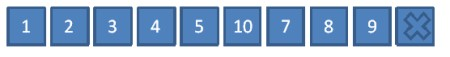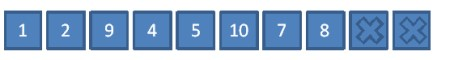# generating a unique range of numbers

Often at work I find the need to generate a set of `n` unique integers in a specified range. In order to do this as efficiently and as easily as possible, I created a small C# class, which a colleague thought may be of general interest. Hence, I’m posting it here. I’m sure someone else has come up with a similar (or better) way to do this in the past, but I’m sharing my way regardless 😀 .

The code is shown below (and you download the C# class here):

```1 2 3 4 5 6 7 8 9 10 11 12 13 14 15 16 17 18 19 20 21 22 23 24 25 26 27 28 29 30 31 32 33 34 35 class UniqueSetGenerator { private int[] store_; private int size_; private Random random_;   public UniqueSetGenerator(int size, int start) { size_ = size; store_ = new int[size]; random_ = new Random(); PopulateArray(start); }   private void PopulateArray(int start) { for (int i = 0; i < size_; i++) store_[i] = start++; }   private int Delete(int pos) { int val = store_[pos]; store_[pos] = store_[--size_]; return val; }   public int GetRandomNumber() { if (size_ <= 0) return -1;   return Delete(random_.Next(size_)); } }```

I think it’s pretty easy to see how this works, so I will not go into it in much detail discussing it, but the sequence of figures below shows the basic operations.First populate the array with the values.Use the `Random` class to obtain our first random number - in this case 6. Now copy the value at position `size_` in the array to position 6, and decrement `size_`. Note: not we don't actually delete anything from the array.We then use the `Random `class to generate another random number in our reduced range - in this case 3. We then copy the value at position `size_ `(i.e. 9) to position 3, and then decrement the `size_ `count as before. This process continues until size_ is reduced to 0.

So when would you require something like this? Well, say you need to generate unique random IDs with values 1 to 10, then you can use the class as follows:

```1 2 3 4 5 6 7 8 UniqueSetGenerator uniqueSet = new UniqueSetGenerator(10, 1);   for(int i = 0; i < 9; i++) { int id = uniqueSet.GetRandomNumber();   // Now do something with the id.... }```

For the moment I have only included a C# version but I will update this post with a Java version soon. Hope some of you find this useful.